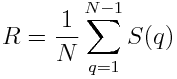# Measuring graph robustness

There are a couple ways to measure how well a graph remains connected when nodes are removed. One ways is to consider nodes dropping out randomly. Another way, the one we look at here, assumes an adversary is trying to remove the most well-connected nodes. This approach was defined by Schneider et al . It is also described, a little more clearly, in .

The robustness of a graph is defined asThen  explains that

N is the total number of nodes in the initial network and S(q) is the relative size of the largest connected component after removing q nodes with the highest degrees. The normalization factor 1/N ensures 1/NR ≤ 0.5. Two special cases exist: R = 1/N corresponds to star-like networks while R = 0.5 represents the case of the fully connected network.

Apparently “relative size” means relative to the size of the original network, not to the network with q nodes removed.

There is one difference between  and . The former defines the sum up to N and the latter to N-1. But this doesn’t matter because S(N) = 0: when you remove N nodes from a graph with N nodes, there’s nothing left!

I have a couple reservations. One is that it’s not clear whether R is well defined.

Consider the phrase “removing q nodes with the highest degrees.” What if you have a choice of nodes with the highest degree? Maybe all nodes have degree no more than n and several have degree exactly n. It seems your choice of node to remove matters. Removing one node of degree n may give you a very different network than removing another node of the same degree.

Along the same lines, it’s not clear whether it matters how one removes more than one degree. For S(2), for example, does it matter whether I remove the two most connected nodes from the original graph at once, or remove the most connected node first, then the most connected node from what remains?

My second reservation is that I don’t think the formula above gives exactly the extreme values it says. Start with a star graph. Once you remove the center node, there are only isolated points left, and each point is 1/N of the original graph. So all the S(q) are equal to 1/N. Then R equals (N – 1)/ N2, which is less than 1/N.

With the complete graph, removing a point leaves a complete graph of lower order. So S(q) = (Nq)/N. Then R equals (N – 1)/2N, which is less than 1/2.CM Schneider et al, Mitigation of malicious attacks on networks. P. Natl. Acad. March 8, 2011, vol. 108. no. 10

 Big Data of Complex Networks, CRC Press. Matthias Dehmer et al editors.

## 2 thoughts on “Measuring graph robustness”

1. The low-power mesh (packet relay) networking community has had to deal with such problems for decades.

While graph theory provided many insights, brute-force modeling was the standard approach to determining network robustness. I’m not at all sure if that’s still the case in current practice.

2. I also don’t see why it is justified to remove only high degree nodes. There might exist some low degree nodes whose removal greatly disconnects/disrupts the graph. The high degree idea is just a heuristic approach for doing this, and I don’t see why this particular heuristic for disconnecting a graph should be THE definition for graph robustness.